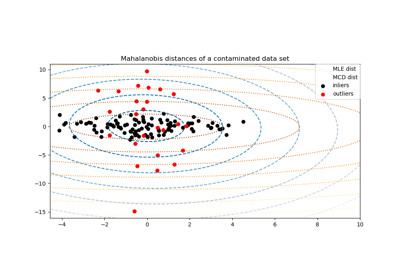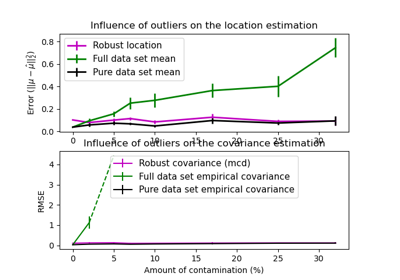# sklearn.covariance.MinCovDet¶

class sklearn.covariance.MinCovDet(*, store_precision=True, assume_centered=False, support_fraction=None, random_state=None)[source]

Minimum Covariance Determinant (MCD): robust estimator of covariance.

The Minimum Covariance Determinant covariance estimator is to be applied on Gaussian-distributed data, but could still be relevant on data drawn from a unimodal, symmetric distribution. It is not meant to be used with multi-modal data (the algorithm used to fit a MinCovDet object is likely to fail in such a case). One should consider projection pursuit methods to deal with multi-modal datasets.

Read more in the User Guide.

Parameters:
store_precisionbool, default=True

Specify if the estimated precision is stored.

assume_centeredbool, default=False

If True, the support of the robust location and the covariance estimates is computed, and a covariance estimate is recomputed from it, without centering the data. Useful to work with data whose mean is significantly equal to zero but is not exactly zero. If False, the robust location and covariance are directly computed with the FastMCD algorithm without additional treatment.

support_fractionfloat, default=None

The proportion of points to be included in the support of the raw MCD estimate. Default is None, which implies that the minimum value of support_fraction will be used within the algorithm: (n_sample + n_features + 1) / 2. The parameter must be in the range (0, 1).

random_stateint, RandomState instance or None, default=None

Determines the pseudo random number generator for shuffling the data. Pass an int for reproducible results across multiple function calls. See Glossary.

Attributes:
raw_location_ndarray of shape (n_features,)

The raw robust estimated location before correction and re-weighting.

raw_covariance_ndarray of shape (n_features, n_features)

The raw robust estimated covariance before correction and re-weighting.

raw_support_ndarray of shape (n_samples,)

A mask of the observations that have been used to compute the raw robust estimates of location and shape, before correction and re-weighting.

location_ndarray of shape (n_features,)

Estimated robust location.

covariance_ndarray of shape (n_features, n_features)

Estimated robust covariance matrix.

precision_ndarray of shape (n_features, n_features)

Estimated pseudo inverse matrix. (stored only if store_precision is True)

support_ndarray of shape (n_samples,)

A mask of the observations that have been used to compute the robust estimates of location and shape.

dist_ndarray of shape (n_samples,)

Mahalanobis distances of the training set (on which fit is called) observations.

n_features_in_int

Number of features seen during fit.

New in version 0.24.

feature_names_in_ndarray of shape (n_features_in_,)

Names of features seen during fit. Defined only when X has feature names that are all strings.

New in version 1.0.

EllipticEnvelope

An object for detecting outliers in a Gaussian distributed dataset.

EmpiricalCovariance

Maximum likelihood covariance estimator.

GraphicalLasso

Sparse inverse covariance estimation with an l1-penalized estimator.

GraphicalLassoCV

Sparse inverse covariance with cross-validated choice of the l1 penalty.

LedoitWolf

LedoitWolf Estimator.

OAS

Oracle Approximating Shrinkage Estimator.

ShrunkCovariance

Covariance estimator with shrinkage.

References

[Rouseeuw1984]

P. J. Rousseeuw. Least median of squares regression. J. Am Stat Ass, 79:871, 1984.

[Rousseeuw]

A Fast Algorithm for the Minimum Covariance Determinant Estimator, 1999, American Statistical Association and the American Society for Quality, TECHNOMETRICS

[ButlerDavies]

R. W. Butler, P. L. Davies and M. Jhun, Asymptotics For The Minimum Covariance Determinant Estimator, The Annals of Statistics, 1993, Vol. 21, No. 3, 1385-1400

Examples

>>> import numpy as np
>>> from sklearn.covariance import MinCovDet
>>> from sklearn.datasets import make_gaussian_quantiles
>>> real_cov = np.array([[.8, .3],
...                      [.3, .4]])
>>> rng = np.random.RandomState(0)
>>> X = rng.multivariate_normal(mean=[0, 0],
...                                   cov=real_cov,
...                                   size=500)
>>> cov = MinCovDet(random_state=0).fit(X)
>>> cov.covariance_
array([[0.7411..., 0.2535...],
[0.2535..., 0.3053...]])
>>> cov.location_
array([0.0813... , 0.0427...])


Methods

 Apply a correction to raw Minimum Covariance Determinant estimates. error_norm(comp_cov[, norm, scaling, squared]) Compute the Mean Squared Error between two covariance estimators. fit(X[, y]) Fit a Minimum Covariance Determinant with the FastMCD algorithm. get_params([deep]) Get parameters for this estimator. Getter for the precision matrix. Compute the squared Mahalanobis distances of given observations. Re-weight raw Minimum Covariance Determinant estimates. score(X_test[, y]) Compute the log-likelihood of X_test under the estimated Gaussian model. set_params(**params) Set the parameters of this estimator.
correct_covariance(data)[source]

Apply a correction to raw Minimum Covariance Determinant estimates.

Correction using the empirical correction factor suggested by Rousseeuw and Van Driessen in [RVD].

Parameters:
dataarray-like of shape (n_samples, n_features)

The data matrix, with p features and n samples. The data set must be the one which was used to compute the raw estimates.

Returns:
covariance_correctedndarray of shape (n_features, n_features)

Corrected robust covariance estimate.

References

[RVD]

A Fast Algorithm for the Minimum Covariance Determinant Estimator, 1999, American Statistical Association and the American Society for Quality, TECHNOMETRICS

error_norm(comp_cov, norm='frobenius', scaling=True, squared=True)[source]

Compute the Mean Squared Error between two covariance estimators.

Parameters:
comp_covarray-like of shape (n_features, n_features)

The covariance to compare with.

norm{“frobenius”, “spectral”}, default=”frobenius”

The type of norm used to compute the error. Available error types: - ‘frobenius’ (default): sqrt(tr(A^t.A)) - ‘spectral’: sqrt(max(eigenvalues(A^t.A)) where A is the error (comp_cov - self.covariance_).

scalingbool, default=True

If True (default), the squared error norm is divided by n_features. If False, the squared error norm is not rescaled.

squaredbool, default=True

Whether to compute the squared error norm or the error norm. If True (default), the squared error norm is returned. If False, the error norm is returned.

Returns:
resultfloat

The Mean Squared Error (in the sense of the Frobenius norm) between self and comp_cov covariance estimators.

fit(X, y=None)[source]

Fit a Minimum Covariance Determinant with the FastMCD algorithm.

Parameters:
Xarray-like of shape (n_samples, n_features)

Training data, where n_samples is the number of samples and n_features is the number of features.

yIgnored

Not used, present for API consistency by convention.

Returns:
selfobject

Returns the instance itself.

get_params(deep=True)[source]

Get parameters for this estimator.

Parameters:
deepbool, default=True

If True, will return the parameters for this estimator and contained subobjects that are estimators.

Returns:
paramsdict

Parameter names mapped to their values.

get_precision()[source]

Getter for the precision matrix.

Returns:
precision_array-like of shape (n_features, n_features)

The precision matrix associated to the current covariance object.

mahalanobis(X)[source]

Compute the squared Mahalanobis distances of given observations.

Parameters:
Xarray-like of shape (n_samples, n_features)

The observations, the Mahalanobis distances of the which we compute. Observations are assumed to be drawn from the same distribution than the data used in fit.

Returns:
distndarray of shape (n_samples,)

Squared Mahalanobis distances of the observations.

reweight_covariance(data)[source]

Re-weight raw Minimum Covariance Determinant estimates.

Re-weight observations using Rousseeuw’s method (equivalent to deleting outlying observations from the data set before computing location and covariance estimates) described in [RVDriessen].

Parameters:
dataarray-like of shape (n_samples, n_features)

The data matrix, with p features and n samples. The data set must be the one which was used to compute the raw estimates.

Returns:
location_reweightedndarray of shape (n_features,)

Re-weighted robust location estimate.

covariance_reweightedndarray of shape (n_features, n_features)

Re-weighted robust covariance estimate.

support_reweightedndarray of shape (n_samples,), dtype=bool

A mask of the observations that have been used to compute the re-weighted robust location and covariance estimates.

References

A Fast Algorithm for the Minimum Covariance Determinant Estimator, 1999, American Statistical Association and the American Society for Quality, TECHNOMETRICS

score(X_test, y=None)[source]

Compute the log-likelihood of X_test under the estimated Gaussian model.

The Gaussian model is defined by its mean and covariance matrix which are represented respectively by self.location_ and self.covariance_.

Parameters:
X_testarray-like of shape (n_samples, n_features)

Test data of which we compute the likelihood, where n_samples is the number of samples and n_features is the number of features. X_test is assumed to be drawn from the same distribution than the data used in fit (including centering).

yIgnored

Not used, present for API consistency by convention.

Returns:
resfloat

The log-likelihood of X_test with self.location_ and self.covariance_ as estimators of the Gaussian model mean and covariance matrix respectively.

set_params(**params)[source]

Set the parameters of this estimator.

The method works on simple estimators as well as on nested objects (such as Pipeline). The latter have parameters of the form <component>__<parameter> so that it’s possible to update each component of a nested object.

Parameters:
**paramsdict

Estimator parameters.

Returns:
selfestimator instance

Estimator instance.

## Examples using sklearn.covariance.MinCovDet¶Robust covariance estimation and Mahalanobis distances relevance

Robust covariance estimation and Mahalanobis distances relevanceRobust vs Empirical covariance estimate

Robust vs Empirical covariance estimate